• 是可以实现线性计算的，numpy库提供了矩阵运算，可以实现求取矩阵特征向量，scipy也可以实现numpy库提供的矩阵运算功能，是对numpy库提供矩阵运算的扩展，本文介绍python中计算矩阵特征向量的两种方法：1、使用numpy...python作为编程中较为简单的编程方法，是可以实现线性计算的，numpy库提供了矩阵运算，可以实现求取矩阵特征向量，scipy也可以实现numpy库提供的矩阵运算功能，是对numpy库提供矩阵运算的扩展，本文介绍python中计算矩阵特征向量的两种方法：1、使用numpy.linalg.eig(a)函数；2、使用scipy.linalg.eig()计算方阵的特征向量(numpy方法的拓展)。一、使用numpy.linalg.eig(a)函数参数:a：想要计算奇异值和右奇异值的方阵。返回值：w：特征值。每个特征值根据它的多重性重复。这个数组将是复杂类型，除非虚数部分为0。当传进的参数a是实数时，得到的特征值是实数。v：特征向量。使用实例>>> from numpy import linalg as LA>>> a = np.array([[1, 1j], [-1j, 1]])>>> w, v = LA.eig(a)>>> w; varray([  2.00000000e+00+0.j,   5.98651912e-36+0.j]) # i.e., {2, 0}array([[ 0.00000000+0.70710678j,  0.70710678+0.j        ],[ 0.70710678+0.j        ,  0.00000000+0.70710678j]])>>> a = np.array([[1 + 1e-9, 0], [0, 1 - 1e-9]])>>> # Theor. e-values are 1 +/- 1e-9>>> w, v = LA.eig(a)>>> w; varray([ 1.,  1.])array([[ 1.,  0.],[ 0.,  1.]])二、使用scipy.linalg.eig()计算方阵的特征向量(numpy方法的拓展)1、语法格式print('Eig:',lg.eig(arr)) #求矩阵arr的特征向量2、使用实例#coding:utf-8from __future__ import divisionfrom scipy import linalg as lafrom scipy import optimizeimport sympyimport numpy as npsympy.init_printing()import matplotlib.pyplot as plt# 使用scipy求解矩阵特征值A = np.array([[1, 3, 5], [3, 5, 3], [5, 3, 9]])evals, evecs = la.eig(A)eigvalues = la.eigvalsh(A)以上就是python中计算矩阵特征向量的两种方法，希望能对你有所帮助哟~
展开全文• 旋转矩阵也叫方向余弦旋转矩阵是一个 3 x3 阶的矩阵，矩阵的列表示载体坐标系中的单位矢量在参考坐标系中的投影。2. 旋转向量也叫轴角任意旋转都可以用一个旋转轴和一个旋转角刻画。旋转向量可以由罗德里格斯公式...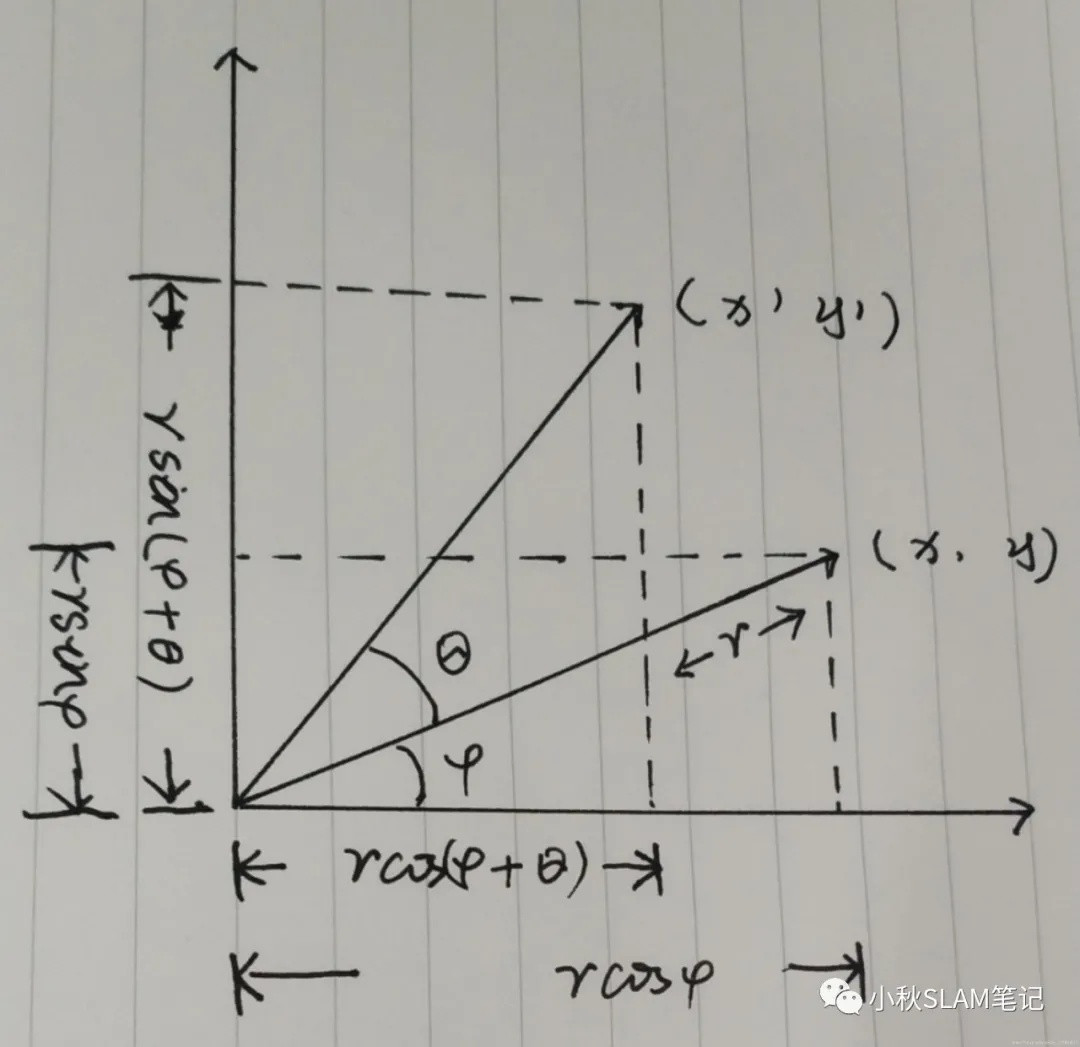姿态的四种表达方式1. 旋转矩阵也叫方向余弦旋转矩阵是一个 3 x3 阶的矩阵，矩阵的列表示载体坐标系中的单位矢量在参考坐标系中的投影。2. 旋转向量也叫轴角任意旋转都可以用一个旋转轴和一个旋转角刻画。旋转向量可以由罗德里格斯公式转换成旋转矩阵3. 欧拉角从一个坐标系到另一个坐标系的变换可以通过依次绕不同坐标轴的3次连续转动来定义，从物理角度看欧拉角表示法可能是最简单的方法之一。4. 四元数四元数姿态表示法，通过绕参考坐标系中两个矢量的单次转动来实现一个坐标系到另一个坐标系的转换。四元数是一个具有四个元素的矢量表达式，各个元素为矢量方向和转动大小的函数。旋转矩阵(方向余弦)旋转矩阵的推倒过程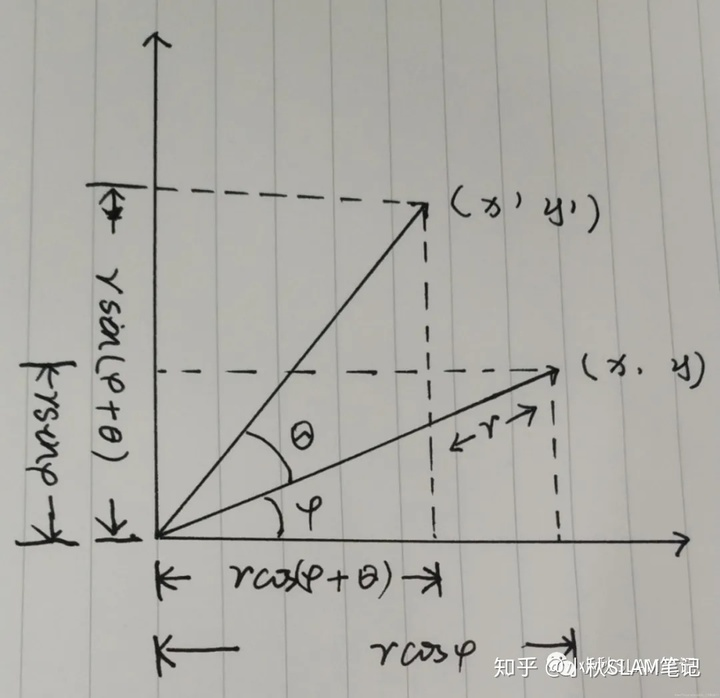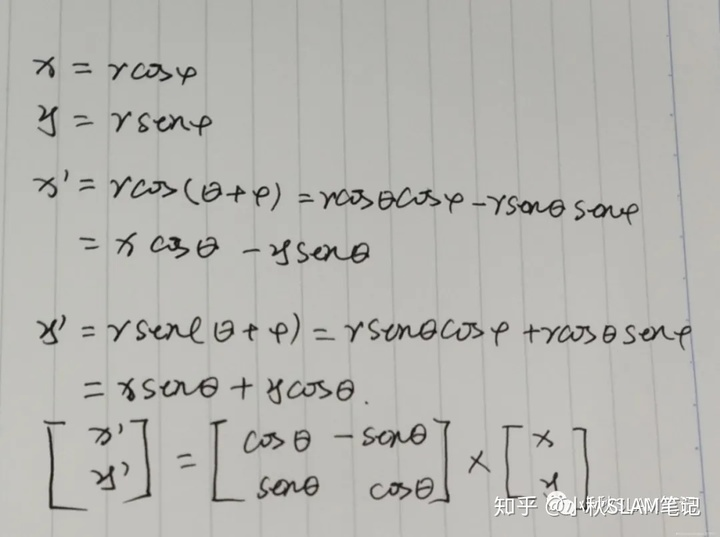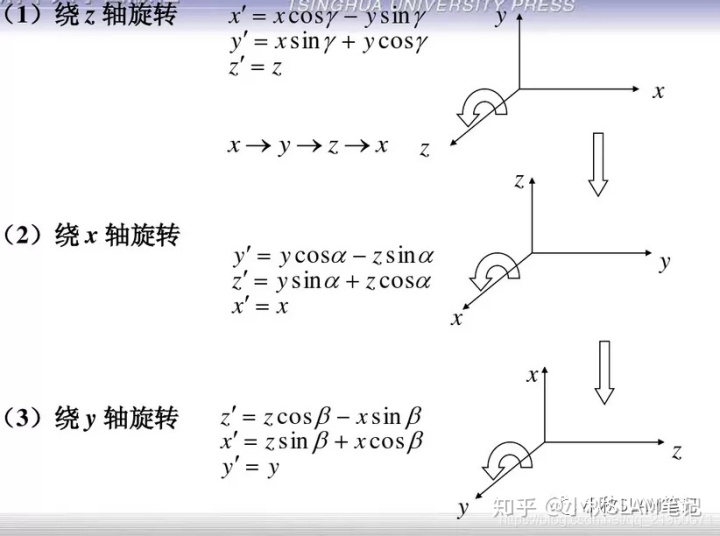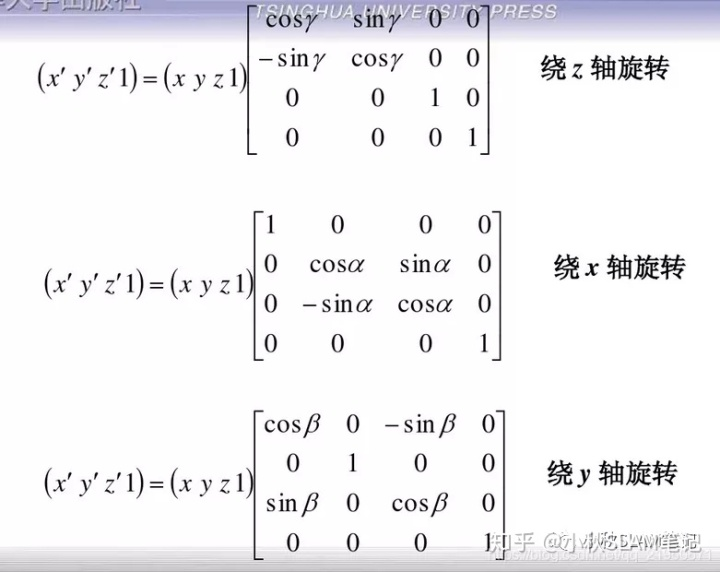Eigen中的旋转矩阵//  旋转矩阵
Eigen::Matrix3d rotation_matrix;
// 绕 Z 轴旋转 45 度的旋转矩阵 sin45 = cos45 = 0.707107
rotation_matrix << 0.707107, -0.707107, 0,
0.707107,  0.707107, 0,
0,         0,        1;
std::cout << "rotation_matrix =n" << rotation_matrix << std::endl;
/*
* rotation_matrix =
* 0.707107   -0.707107   0
* 0.707107    0.707107    0
* 0           0         1
*/
Eigen中的旋转向量(轴角)// 旋转矩阵转换为旋转向量
Eigen::AngleAxisd rotation_vector;
rotation_vector.fromRotationMatrix(rotation_matrix);
std::cout << "rotation_vector " << "angle is: " << rotation_vector.angle() << " axis is: "
<< rotation_vector.axis().transpose() << std::endl;
/*
* rotation_vector angle is: 0.785398 axis is: 0 0 1
*/
// 弧度转角
cout << 0.785398 * 180 / M_PI << endl; // 45
欧拉角欧拉角是描述刚体姿态的三个角，欧拉角有静态和动态两种，静态的是绕静止的惯性坐标系三个轴进行旋转，而动态的在旋转过程中旋转坐标轴会发生变化，除了第一次旋转是绕惯性系的坐标轴进行之外，后续两次旋转都是动态的，并且前面旋转的角度对后面的旋转轴是有影响的，按照不同的轴顺序进行旋转得到的欧拉角也是不同的，旋转变换可以归结为若干个沿着坐标轴旋转的组合，组合个数不超过三个并且两个相邻的旋转必须沿着不同坐标轴，总共有12种旋转方式，分别是XYZ、XZY、XYX、XZX、YXZ、YZX、YXY、YZY、ZXY、ZYX、ZXZ、ZYZ。ROS里面URDF采用的是XYZ(rpy)，规定右手坐标系中，物体旋转的正方向是右手螺旋方向。 在右手直角坐标系中，从原点看，沿每一根轴的顺时针方向定义为这根轴的正向转动，负向转动正好相反，即为逆时针方向。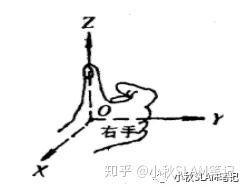必须记住的是，当绕不同的轴系作一系列转动时，载体姿态的变化不仅是绕每根轴转动角度的函数，而且还是转动顺序的函数。左半图转动的顺序:先绕俯仰轴 y 转动 90 再绕偏航轴 z 转动 90 最后绕俯仰轴 y 转动 90 依次完成转动后，可以看到绕横滚轴 x 发生了 90 的净转动。右半图中转动的顺序正好相反虽然转动结束时，载体的横滚轴仍对准在原来的方向，但横滚轴 x 发生了 -90 的净转动。各个轴的转动顺序是不可交换的。很明显，如不考虑轴系的转动顺序，在计算姿态时将会引起很大的误差。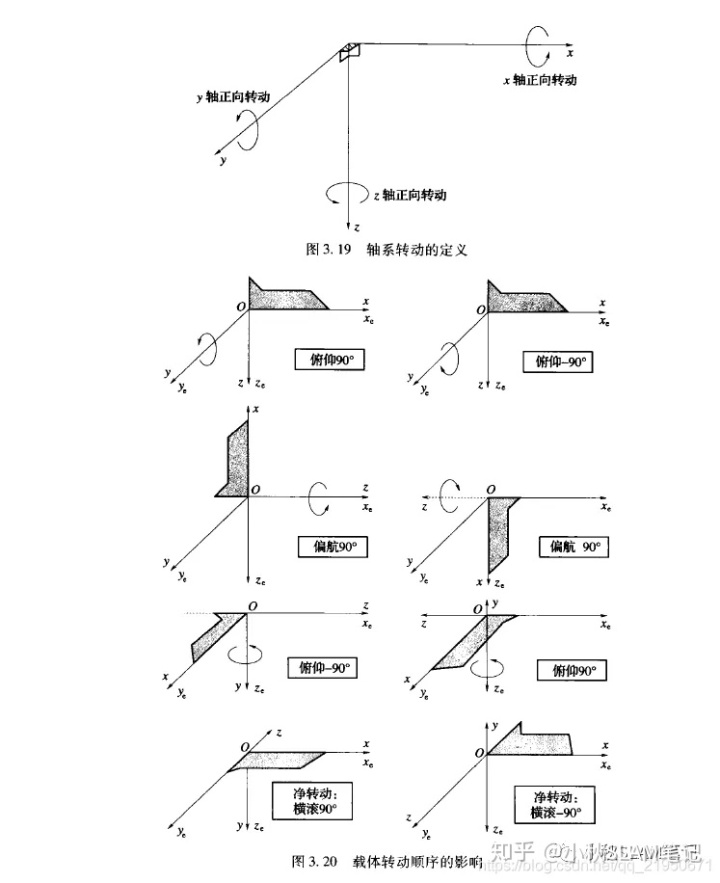Eigen中的欧拉角// 旋转矩阵转换为欧拉角
// "2" represents the z axis and "0" the x axis "1" the y axis
// 动轴: ZYX顺序,即先绕x轴roll旋转,再绕y轴pitch旋转,最后绕z轴yaw旋转,
Eigen::Vector3d euler_angles = rotation_matrix.eulerAngles(2, 1, 0);
// 默认向量都是列向量，所以为了书写和显示方便，转置成行向量
std::cout << "yaw(z) pitch(y) roll(x) = " << euler_angles.transpose() << std::endl;
/*
*  yaw(z) pitch(y) roll(x) = 0.785398  -0  0
*/
cout << 0.785398 * 180 / M_PI << endl; // 45
Eigen中的四元数// 找不到不带奇异性的三维向量描述方式，四元数是紧凑的，没有奇异性
// 旋转矩阵转换为四元数
Eigen::Quaterniond quaterniond = Eigen::Quaterniond(rotation_matrix);
std::cout << "quaternion from rotation matrix = n" << quaterniond.coeffs() << std::endl;
/*
* quaternion from rotation matrix =
* 0
* 0
* 0.382684
* 0.92388
*/
std::cout << "quaterniond x: " << quaterniond.x() << std::endl;
std::cout << "quaterniond y: " << quaterniond.y() << std::endl;
std::cout << "quaterniond z: " << quaterniond.z() << std::endl;
std::cout << "quaterniond w: " << quaterniond.w() << std::endl;
/*
* quaternion2 x: 0
* quaternion2 y: 0
* quaternion2 z: 0.382684
* quaternion2 w: 0.92388
*/
四种位姿可视化视觉SLAM十四讲实践笔记之四种位姿可视化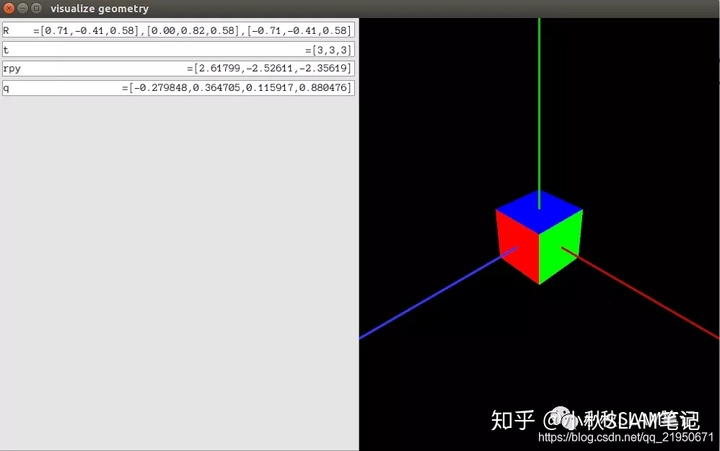变换矩阵// 变换矩阵
Eigen::Isometry3d transform_matrix = Eigen::Isometry3d::Identity();
transform_matrix.rotate(rotation_matrix);
transform_matrix.pretranslate(Eigen::Vector3d(1, 3, 4));
std::cout << "Transform matrix = n" << transform_matrix.matrix() << std::endl;
/*
* Transform matrix =
* 0.707107 -0.707107         0         1
* 0.707107  0.707107         0         3
*        0         0         1         4
*        0         0         0         1
*/
用变换矩阵进行坐标变换 视觉SLAM十四讲实践之坐标系之间的欧式变换// 坐标变换
Eigen::Vector3d v_src(1, 0, 0);
Eigen::Vector3d v_tar;
v_tar = transform_matrix * v_src;
std::cout << "(1,0,0) after rotation = " << v_tar.transpose() << std::endl;
// (1,0,0) after rotation = 1.70711 3.70711  4
位姿的估计和优化视觉SLAM十四讲实践之Sophus下的位姿表示和更新优化点击下方阅读原文可以获得更好阅读体验，并且会对原文进行迭代更新；欢迎和我一起交流学习；所有内容来自网络如有侵权请联系删除；仅代表个人观点，不喜勿喷！阅读原文
展开全文• tensorflow的表示类似于numpy，习惯python表达，容易将向量矩阵弄混，记录下两者区别 case1: 对于numpy矩阵： numpy和tensor表达如下： A = np.array([[1,-1,2], [3,2,0]]) A_tf = tf.constan([[1,-1,2], [3...

tensorflow的表示类似于numpy，习惯python的表达，容易将向量和矩阵弄混，记录下两者区别

case1: 对于numpy矩阵：numpy和tensor的表达如下：
A = np.array([[1,-1,2], [3,2,0]])
A_tf = tf.constan([[1,-1,2], [3,2,0]])

注意是两对中括号，少了一对则是向量
case2： 单列的数组，构建方法numpy和tensor的表达如下：
A = np.array([, , ])
A_tf = tf.constan([, , ])

也可以通过行向量转置获得相关列向量
v = np.transpose(np.array([[2,1,3]]))


具体的一些numpy公式计算可以参考：
https://www.numpy.org.cn/article/basics/numpy_matrices_vectors.html#%E5%BA%94%E7%94%A8%EF%BC%9A%E5%A4%9A%E5%85%83%E7%BA%BF%E6%80%A7%E5%9B%9E%E5%BD%92


展开全文• 1.矩阵的几种表示方法 &gt;&gt; A=[1 2 3;4 5 6;7 8 9]//例如1和2之间可以是空格，也可以是逗号 A =    1 2 3  4 5 6  7 8 9   &gt;&gt; B=[-1 -2 -3;-4 -5 -6;-7 -8 -9] B = ...
1.4矩阵的表示

1.矩阵的几种表示方法

>> A=[1 2 3;4 5 6;7 8 9]//例如1和2之间可以是空格，也可以是逗号

A =

1     2     3

4     5     6

7     8     9

>> B=[-1 -2 -3;-4 -5 -6;-7 -8 -9]

B =

-1    -2    -3

-4    -5    -6

-7    -8    -9

>> C=[A B;B A]//矩阵可以组合表示

C =

1     2     3    -1    -2    -3

4     5     6    -4    -5    -6

7     8     9    -7    -8    -9

-1    -2    -3     1     2     3

-4    -5    -6     4     5     6

-7    -8    -9     7     8     9

>> B=[1 2 3;4 5 6]

B =

1     2     3

4     5     6

>> C=[6 7 8;9 10 11]

C =

6     7     8

9    10    11

>> A=B+i*C//从这里可以看出：数值变量*矩阵=数值变量*矩阵中每一个元素

A =

1.0000 + 6.0000i   2.0000 + 7.0000i   3.0000 + 8.0000i

4.0000 + 9.0000i   5.0000 +10.0000i   6.0000 +11.0000i

2.冒号表达式（非常重要）

>> T=0:1:5//初始值：步长：终止值

T =

0     1     2     3     4     5

>> T=0:5//步长默认是1

T =

0     1     2     3     4     5

3.linspace函数

>> X=linspace(0,pi,6)//linspace(初始值, 终止值, 变量个数)

X =

0    0.6283    1.2566    1.8850    2.5133    3.1416

4.结构矩阵

1,2,3是元素，x1,x2,x3是成员变量

>> a(1).x1=10;a(1).x2='liu';a(1).x3=[11 21;34 78];

>> a(2).x1=12;a(2).x2='wang';a(2).x3=[34 191;27 578];

>> a(3).x1=14;a(3).x2='cai';a(3).x3=[13 890;67 231];

>> a

a =

1x3 struct array with fields:

x1

x2

x3

1.5矩阵元素的引用

1.A(x,y)和A(x)

A=[1 2 3;4 5 6];

>> A(4,5)=10//没有（4,5）这个元素，但是不会报错

A =

1     2     3     0     0

4     5     6     0     0

0     0     0     0     0

0     0     0     0    10

>> A(3) //A(3)中的3是指序列号（按列），m*n的矩阵对应序列号是（j-1）*m+i

ans =

0

>> A(10)

ans =

6

2. A(x,y)和A(x)的转化

>> A=[1:3;4:6]//此处用了冒号表达式

A =

1     2     3

4     5     6

>> D=sub2ind(size(A),[1 2;2 2],[1 1;3 2])// sub2ind：将下标转化成序列号

D =

1     2

6     4

>> [i,j]=ind2sub(size(A),2)//将序列号转换成下标

i =

2

j =

1

3 .冒号表达式引用矩阵元素

>> A=[1 2 3 4 5;6 7 8 9 10;11 12 13 14 15]

A =

1     2     3     4     5

6     7     8     9    10

11    12    13    14    15

>> A(1:2,:)//：表示全体

ans =

1     2     3     4     5

6     7     8     9    10

>> A(2:3,1:2:5)//1:2:5代表第1,3,5列

ans =

6     8    10

11    13    15

>> A=[1 2 3 4 5;6 7 8 9 10;11 12 13 14 15;16 17 18 19 20];

>> A(end,:)//end代表最后一行或者列

ans =

16    17    18    19    20

4.删除矩阵元素

>> A=[]//将元素全部删除

A =

[]

>> A=[1 2 3 0 0;7 0 9 2 6;1 4 -1 1 8]

A =

1     2     3     0     0

7     0     9     2     6

1     4    -1     1     8

>> A(:,[2,4])=[]//将第2,4列的全部元素删除

A =

1     3     0

7     9     6

1    -1     8

5.改变矩阵的m*n=p*q

>> B=reshape(A,1,9)

B =

1     7     1     3     9    -1     0     6     8

>> C=A(:)//代表将元素在一列表示出来，在理解下标和序列号时用

C =

1

7

1

3

9

-1

0

6

8


展开全文MATLAB
• 三维向量的叉积 令x1\mathbf x_1 = (x1,y1,t1)T\begin{pmatrix} x_1 , y_1 , t_1\end{pmatrix}^T , x2\mathbf x_2 = (x2,y2,t2)T\begin{pmatrix} x_2 , y_2 , t_2\end{pmatrix}^T 是两个三维向量，它们的叉积定义为...计算机视觉
• 旋转矩阵也叫方向余弦旋转矩阵是一个 3 x3 阶的矩阵，矩阵的列表示载体坐标系中的单位矢量在参考坐标系中的投影。2. 旋转向量也叫轴角任意旋转都可以用一个旋转轴和一个旋转角刻画。旋转向量可以由罗德里格斯公式...
• 在进行图像处理时往往会遇到需要将矩阵表示的特征或者灰度值转化为向量形式，以下是使用两套方案。 在此不区分行/列向量，可以用“ ’ ”转置就好 方案一： A = A(: ); clc; clear; A = [1 2 3;4 5 6 ;7 8 9]; ...
• 在机器学习的各种关于向量或者矩阵的求导中，经常会出现各种需要转置或者不需要转置的地方，经常会看得人晕头转向。...其中，分子的标量形式为 ，分子的向量形式表示为 ，分子的矩阵形式表示为 ；类似的，分母的标量...
• 矩阵的特征值和特征向量方法 第一步我们首先需要知道计算矩阵的特征值和特征向量要用eig函数，可以在命令行窗口中输入help eig，查看一下eig函数用法 第二步在命令行窗口中输入a=[1 2 3;2 4 5;7 8 9]，按回车键...
• python中运算符有哪些算术运算符比较(关系)运算符赋值运算符逻辑运算符位运算符成员运算符身份运算符运算符优先级如何使用python表示矩阵使python表示矩阵的方法：使用“import numpy”语入numpy包。用numpy包...
• 目标：本文讨论单词向量的组合方式（composition of word vectors），一般指两个单词向量的组合方式，使得输出的...R：一个表征语法关系的矩阵； K：世界知识等其他非单词语义、语法关系构成的信息； 词语组合的一些假
• 矩阵的乘法有3种不同的方法去解释它们意义，而这三种方法都是等效。 分别是： 坐标空间变换 跃迁运动 方法乘以对象 引子： 二维平面上有A、B两个点。 很明显，对于这两个点，我们可以任意建立坐标系；而不同...
• 6.6.1 向量范数 约定：用 表示所有 n 维实向量 实线性空间。 在 上引入向量范数定义如下： 定义6.2： 上的向量范数是定义在 某个实值函数 ，它满足如下三个条件： 6.6 向量范数和矩阵范数 (1)||x||?0，...
• NumPy 中的矩阵向量numpy的ndarray类用于表示矩阵和向量。要在numpy中构造矩阵，我们在列表中列出矩阵的行，并将该列表传递给numpy数组构造函数。例如，构造与矩阵对应的numpy数组我们会这样做A = np.array([[1,-1...
• MATLAB向量的创建 1、行向量 行向量有两种表示方法 %示例1 R1 = [1 2 3 4 5] R2 = [1,3,5,7,9] R = R1 + R2 %结果1 R1 = 1 2 3 4 5 R2 = 1 3 ...matlab
• 推导过程中万能的验证方法：即对矩阵/向量的每个分量逐元素求导，再合成矩阵/向量；但往往比较麻烦，故用于验证推导的结果是否正确；变量为向量时，仅将其看作多个实数，无所谓行/列向量之分，有些地方规定结果形如...
• 表示欧几里得向量范数，...MNX1维列向量的意思：维度为MN维列向量，其中该列向量的前M行元素等于图像的第一列元素，令接着的M行元素等于图像的第二列，以此类推。（使用这种方法可以表达适用图像更广泛的线性处理） ...
• 在计算反向传播或最优化问题时，经常遇到向量矩阵、张量对向量矩阵、张量求导问题，而类比普通函数求导经常无法处理矩阵转置问题，因此需要使用一套更简单符号系统进行运算，即里奇微积分。爱因斯坦求和...
• 2. 词袋模型(Bag-of-words)Bag-of-words模型是信息检索领域常用文档表示方法。在文本特征生成过程中，对于一个文档，词袋模型忽略其单词顺序和语法、句法等要素，将其仅仅看作是若干个词汇集合，文档中每个单词...
• 1、数据集概念 按照某种格式来创建数据集，是任何数据分析第一步 1、选择一种数据结构来存储 2、将数据输入或导入到这...数据集通常是有数据构成一个矩形数组，行表示记录，列表示属性（字段）。如下面一个病...R语言
• 文章目录A 线性表示B 基与维数C 向量的坐标D 过渡矩阵 A 线性表示 <1> 定义1（线性表示） <2> 定义2（线性相关） <3> 定义3（线性无关） 注： {α1,α2...αn}\{\alpha_1,\alpha_2...\alpha_...
• Bag-of-words模型是信息检索领域常用文档表示方法。在文本特征生成过程中，对于一个文档，词袋模型忽略其单词顺序和语法、句法等要素，将其仅仅看作是若干个词汇集合，文档中每个单词出现都是独立，不依赖于...python 人工智能
• 首先，采用支持矢量机凸二次约束线性规划表示，然后，综合蒙特卡罗方法和不完全Cholesky分解方法，提出一个新矩阵近似算法KMA-α，该算法首先对核矩阵进行蒙特卡洛随机采样，采样后不直接进行奇异值分解，又...
• 坐标转换有很多种方法，不同领域有不同使用习惯。 上两篇文章我们讲了旋转矩阵和欧拉角，可知欧拉角是可以由旋转矩阵转化而来。 那么怎么从欧拉角转化为旋转矩阵呢？欧拉角（Euler angles）与旋转矩阵...
• NumPy库是Python库中用于数据分析，机器学习，科学计算一个主力，它极大地简化了向量矩阵的操作和处理。Python一些主要软件包依赖于NumPy作为其架构基础部分，比如scikit-learn, SciPy, pandas以及 ...
• 这里有旋转向量的两种表示方法： 1）简单的用一个3×1（3行1列，其中逗号表示一行）的向量来表示，该向量的方向就是旋转轴，它的模就是绕轴逆时针旋转的角度。 Eigen::Vector3d w(0.01,0.02,0.03);//w就是初始化的...
• 线代最核心方法就是用秩去刻画问题，秩在方程组判定，向量线性相关无关，向量线性表示问题，向量极大线性无关组判定，向量等价和矩阵等价判断，齐次线性方程组基础解系问题，一个特征...
• 展开全部表示唯一即需要A中的向量不能相互表示，也就是A中的向量线性无关时，由A中向量表示成b时表示方法唯32313133353236313431303231363533e58685e5aeb931333433643061一。条件：等价于AX=b这个方程有解。要理解一......